Preston College Cosmic Ray Group

Cosmic ray muons and relativistic time dilation

Cosmic ray muons are created when highly energetic particles from deep space collide with atoms in the Earth's upper atmosphere. The initial collisions create pions which then decay into muons. The muons then travel down through the atmosphere and arrive at ground level with a frequency of about 1 cm-2 min-1.

The muon has a measured mean lifetime of about 2.2 microseconds. If the muons travel at nearly the speed of light then the distance traveled in a typical lifetime will be:

s = 2.2 x 10-6 x 3 x 108 m

i.e. a distance of about 660m.

If the muons are produced in the upper atmosphere (15-20 km up) and travel, on average, a distance of about 660m then not many should be capable of reaching the ground. The measured intensity of 1 cm-2 min-1 at ground level is too high according to this calculation.

The high count rate can be explained using relativistic time dilation. Einstein showed that time ticks slowly for particles moving at speeds close to that of light. Whilst the mean lifetime of the muon at rest is of the order microseconds, when it moves at near the speed of light the lifetime is increased by a factor of ten or more. This gives the muons time to reach ground level and accounts for their measured intensity.

The following example of a muon time dilation calculation is based upon the treatment on page 14 of Arnold Wolfendale's comprehensive book 'Cosmic Rays' (published by Newnes, 1963).

We assume that muons are produced at a typical height of about 15km above ground level. If they travel at the speed of light then the time taken to travel the 15 km would be

t = x/c

t = (15 x 103)/(3 x 108)

i.e.   t  = 5 x 10-5 s.

If the mean lifetime of the particles is t = 2.2 x 10-6s then the fraction of muons generated at 15km surviving to reach ground level should be: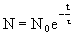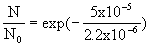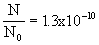This suggests that almost no muons could be expected to reach ground level.

On the other hand, according to the theory of relativity, a particle moving at close to the speed of light should have a mean lifetime of gt instead of the rest lifetime t . The factor g is called the lorentz factor and is given by g = 1/÷ ( 1 - v2/c2) where v is the velocity of the particle and c is the speed of light.

Particle physicists are more likely to work in terms of particle energies rather than velocities and it is useful to derive a value of the lorentz factor from the energy of the particle rather than its velocity.

If we consider 20 GeV muons then we can get g from the Einstein equation E = mc2. This equation can be written in terms of the rest mass of the particle

i.e. E = g m0c2 so g = E/m0c2

In energy terms, the rest mass of the muon is 106MeV so g = 20GeV/106MeV

i.e g = (20 x 109)/(106 x 106)

so g = 189.

The mean lifetime now becomes 189 x 2.2 x 10-6s hence the fraction now capable of reaching ground level becomes: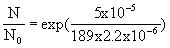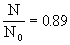This suggests that a significant fraction of the muons created in the upper atmosphere will be capable of reaching ground level. The prediction is consistent with experimental measurements.